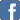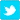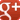# Fun With Numbers - Divisibility Tests

I got a bit lost on YouTube, via Chinese Remainder Theorem and Modular Functions, I ended up with divisibility tests.
Telling by eye whether a number divides by 2, 3, 4, 5, 6, 8, 9 or 10 is pretty straight forward, non-prime numbers like 12 or 15 are also easy enough, you just have to do two tests; if it divides by 3 and by 4, then it must divide by 12 and so on.
There is a neat way of checking whether a long number divides by a prime number, short of actually doing long division. I have no idea what practical purpose this serves, it's just a bit of Fun With Numbers.
You just have to remember the "magic numbers" in this table:
1,-0......11,-1.....21 n/a.....31,-3
3,+1.....13,+4....23,+7..... 33 n/a
7,-2......17,-5.....27 n/a.....37,-11
9,+1.....19,+2....29,+3..... 39 n/a
(21, 33 and 39 are not prime numbers, but for completeness, the entries would be 21,-2; 33,+10; and 39,+4.)
If you can remember the first column, the "magic number" for the prime numbers go up/down by -1 or +1 for numbers ending in 1 or 9, and by +3 or -3 for numbers ending in 3 or 7. So you can reconstruct the whole table just with those two rules, and it goes on forever AFAIAA, so the "magic number" for 73 would be +21. There's no such thing as -0 of course, that's just to help you remember the pattern.
What do we do with the "magic number"?
If you have to work out whether a large number divides by a particular prime, you proceed as follows:
1. Multiply the last digit by the "magic number" and add that to the remaining digits (i.e. subtract it if the "magic number" is negative, or add it if both the "magic number" and the last digit were negative).
2. Do the over and over again until either:
a) you get to zero or a number that divides by the prime you are testing for (pass); or
b) you end up with a number that clearly doesn't divide by the prime you are testing for or the calculation goes circular and starts flipping between positive and negative (fail).
Let's test if 169,682 divides by 37:
16,968 - (11 x 2) = 16,946
1,694 - (11 x 6) = 1,628
162 - (11 x 8) = 74
If it's not obvious to you that 74 = 37 x 2, then keep going...
7 - (11 x 4) = -37
-37 clearly divides by 37, that's a pass.
Let's test if 169,680 divides by 37 (it clearly doesn't):
16,968 - (11 x 0) = 16,968
1,696 - (11 x 8) = 1,608
160 - (11 - 8) = 72
Clearly a fail, but let's keep going...
7 - (11 x 2) = -15
-1 - (11 x -15) = 164
16 - (11 x 4) = -28
-2 - (11 x -8) = 86
At this stage you are flipping from positive to negative, so we can declare this a fail.